# 1.6.2 流密码

author : [email protected]

• 流密码的基本概念
• 线性反馈移位寄存器
• m序列的伪随机性
• m序列密码的破译
• 非线性序列

### 学习目标

• 掌握流密码的基本概念；
• 了解常用伪随机性的评价方法；
• 掌握线性移位寄存器的结构与输出序列的伪随机性；
• 熟悉非线性序列的概念与基本产生方法；
• 掌握一种典型流密码，祖冲之密码或 RC4 密码。

### 流密码的基本概念

#### 2.1.1 二元加法流密码#### 2.1.2 XOR运算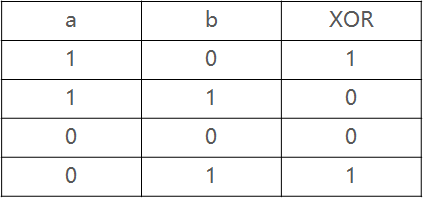XOR有一个特点：如果对一个值连续做两次 XOR，会返回这个值本身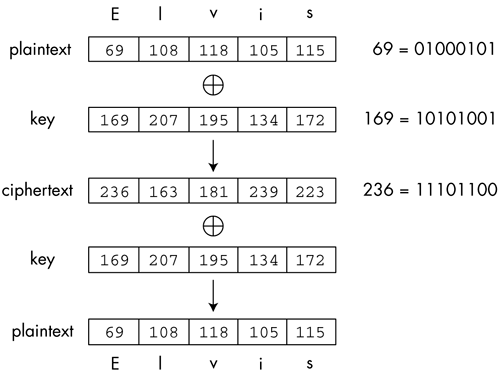#### 2.1.3 密钥序列#### 2.1.4 随机数

• 真正的随机数是使用物理现象产生的：比如掷钱币、骰子、转轮、使用电子元件的噪音、核裂变等等，这样的随机数发生器叫做物理性随机数发生器，它们的缺点是技术要求比较高。
• 使用计算机产生真随机数的方法是获取cpu频率与温度的不确定性以及统计一段时间的运算次数每次都会产生不同的值，系统时间的误差以及声卡的底噪等。
• 在实际应用中往往使用伪随机数就足够了。这些数列是“似乎”随机的数，实际上它们是通过一个固定的、可以重复的计算方法产生的。计算机或计算器产生的随机数有很长的周期性。它们不真正地随机，因为它们实际上是可以计算出来的，但是它们具有类似于随机数的统计特征。这样的发生器叫做伪随机数发生器。

• 统计学伪随机性。统计学伪随机性指的是在给定的随机比特流样本中，1的数量大致等于0的数量，同理，“10”“01”“00”“11”四者数量大致相等。类似的标准被称为统计学随机性。满足这类要求的数字在人类“一眼看上去”是随机的。
• 密码学安全伪随机性。其定义为，给定随机样本的一部分和随机算法，不能有效的演算出随机样本的剩余部分。
• 真随机性。其定义为随机样本不可重现。实际上只要给定边界条件，真随机数并不存在，可是如果产生一个真随机数样本的边界条件十分复杂且难以捕捉（比如计算机当地的本底辐射波动值），可以认为用这个方法演算出来了真随机数。

#### 2.1.5 OTP一次性密码本

• 通过真随机数生成密钥序列
• 每个密钥序列位都只使用一次，也就是和明文等长
• 只有合法的通信方才知道密钥序列，使用后立即销毁

• 最大问题在于密钥的配送
• 密钥的保存
• 密钥的同步
• 密钥的生成

### 通用伪随机数生成器（PRNG）

$$S_0=seed$$

$$S_i+1\equiv f(S_i),i=0,1,...$$

$$S_0=seed$$

$$S_{i+1}\equiv (aS_i+b)\space mod\space m,i=0,1,2,...$$

#### 2.2.1 破译通用伪随机数生成器（PRNG）

PRNG的输出序列非常具有随机性，那我们是否可以用它来生成我们的密钥序列呢？答案是不能，已知明文攻击可以轻松算出PRNG的参数，从而对加密后的明文进行破解。 定义一个线性同余发生器：

$$S_0=seed$$

$$S_{i+1}\equiv (aS_i+b)\space mod\space m,i=0,1,2,...$$

$$y_i\equiv (x_i+s_i)\space mod\space 2$$

$$s_i\equiv (y_i+x_i)\space mod\space m ,i=1,2,...,300$$

$$S_2=(AS_1+B)\space mod\space m$$

$$S_3=(AS_2+B)\space mod\space m$$

$$A\equiv \frac{S_2-S_3}{S_1-S_2}\space mod\space m$$

$$B\equiv \frac{S_1(S_2-S_3)}{S_1-S_2}\space mod\space m$$

$$(a6\space a_7\space a_8\space a_9\space a{10})=(c_5\space c_4\space c_3\space c_2\space c_1) \left{ \begin{matrix} a_1& a_2& a_3& a_4& a_5 \ a_2& a_3& a_4& a_5& a_6 \ a_3& a_4& a_5& a_6& a_7 \ a_4& a_5& a_6& a_7& a_8 \ a_5& a_6& a_7& a_8& a_9 \end{matrix} \right}$$

$$(0\space 1\space 0\space 0\space 0)=\space (c_5\space c_4\space c_3\space c_2\space c_1) \left{ \begin{matrix} 1&1&0&1&0 \ 1&0&1&0&0 \ 0&1&0&0&1 \ 1&0&0&1&0 \ 0&0&1&0&0 \end{matrix} \right}$$

$$\left{ \begin{matrix} 1&1&0&1&0 \ 1&0&1&0&0 \ 0&1&0&0&1 \ 1&0&0&1&0 \ 0&0&1&0&0 \end{matrix} \right}^{-1}= \left{ \begin{matrix} 0&1&0&0&1 \ 1&0&0&1&0 \ 0&0&0&0&1 \ 0&1&0&1&1 \ 1&0&1&1&0 \end{matrix} \right}$$

$$(C_5\space C_4\space C_3\space C_2\space C_1)=(0\space1\space0\space0\space0) \left{ \begin{matrix} 0&1&0&0&1 \ 1&0&0&1&0 \ 0&0&0&0&1 \ 0&1&0&1&1 \ 1&0&1&1&0 \end{matrix} \right}$$

$$(C_5\space C_4\space C_3\space C_2\space C_1)=(1\space 0\space0\space1\space0)$$

$$a{i+5}=c_5a_i\oplus c_2a{i+3}=ai\oplus a{i+3}$$

### 基于位移寄存器的流密码

#### 2.3.1 线性反馈移位寄存器（LFSR）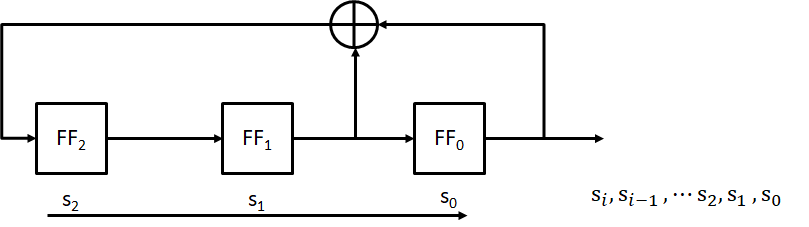#### 2.3.1 线性反馈移位寄存器（LFSR）

$$s_3\equiv (s_1+s_0\space) mod\space 2$$

$$s_4\equiv (s_2+s_1\space) mod\space 2$$

$$s_5\equiv (s_3+s_2\space) mod\space 2$$

$$⋮$$

$$s{i+3}\equiv (s{i+1}+s_i\space) mod\space 2$$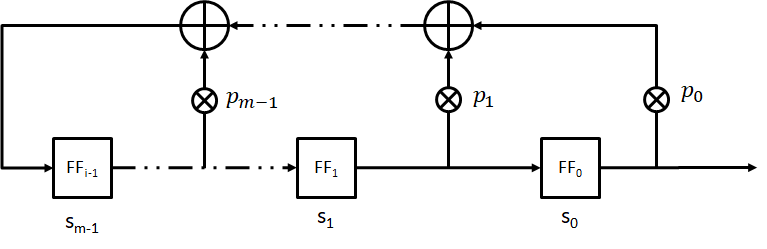$$sm\equiv (s{m-1}p_{m-1}+...+s_1p_1+s_0p_0)\space mod\space 2$$

$$s{i+m}\equiv \sum^{m-1}{j=0}pj\times s{i+j}\space mod\space 2;s_i,p_j\in{0,1};i=0,1,2,...$$

$$p(x)=pnx^n+p{n-1}x^{n-1}+...+p_ix+1$$

#### 2.3.2 破译LFSR流密码

$$s_i\equiv x_i+y_i\space mod \space 2;i=0,1,2,...,2m-1$$

$$i=0,\space sm\equiv s{m-1}p_{m-1}+...+s_1p_1+s_0p_0\space mod2$$

$$i=1,\space s{m+1}\equiv s_mp{m-1}+...+s_2p_1+s_1p_0\space mod2$$

$$i=2,\space s{m+2}\equiv s{m+1}p_{m-1}+...+s_3p_1+s_2p_0\space mod2$$

$$⋮$$

$$i=m-1,\space s{2m-2}p{m-1}+...+smp_1+s{m-1}p_0 \space mod2$$

### 非线性流密码

• 驱动子系统常用一个或多个线性反馈移位寄存器来实现
• 非线性组合子系统用非线性组合函数F来实现

#### 2.4.1 Geffe 序列生成器

Geffe序列生成器由3个LFSR组成，其中LFSR2作为控制生成器使用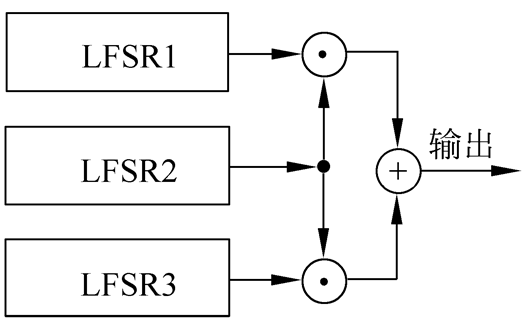$$b_k=a_k^{(1)}a_k^{(2)}+a_k^{(3)}\overline{a_k^{(2)}}=a_k^{(1)}a_k^{(2)}+a_k^{(3)}a_k^{(2)}+a_k^{(3)}$$

Geffe序列生成器也可以表示为图2.13的形式，其中LFSR1和LFSR3作为多路复合器的输入，LFSR2控制多路复合器的输出。设LFSRi的特征多项式分别为ni次本原多项式，且ni两两互素，则Geffe序列的周期为

$$\prod ^{3}_{i=1}(2^{n_i}-1)$$

$$(n_1+n_3)n_2+n_3$$Geffe序列的周期实现了极大化，且0与1之间的分布大体上是平衡的。

#### 2.4.2 J-K触发器

J-K触发器如图所示，它的两个输入端分别用$J(x1)$和$K(x_2)$表示，其输出$c_k$不仅依赖于输入，还依赖于前一个输出位$c{k-1}$，即

$$ck=\overline{(x_1+x_2)}\space c{k-1}+x_1$$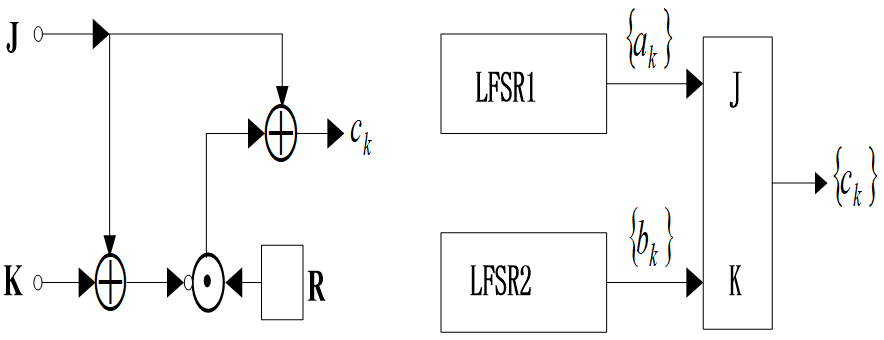$$ck=\overline{a_k+b_k}\space c{k-1}+ak=(a_k+b_k+1)c{k-1}+a_k$$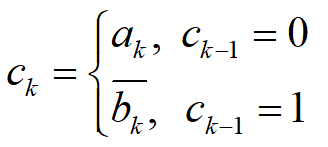#### 2.4.3 Pless生成器

Pless生成器由8个LFSR、4个J-K触发器和1个循环计数器构成，由循环计数器进行选通控制，假定在时刻t输出第t(mod 4)个单元，则输出序列为 a0b1c2d3a4b5d6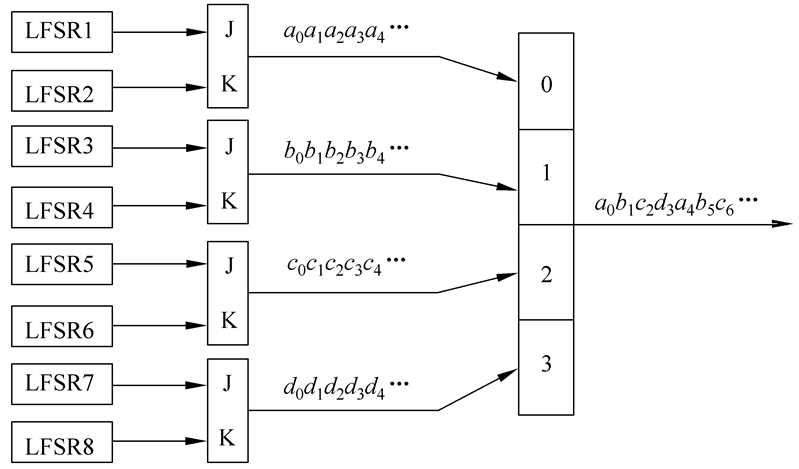#### 2.4.4 钟控序列生成器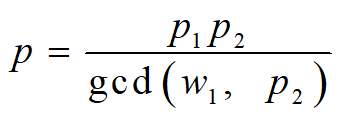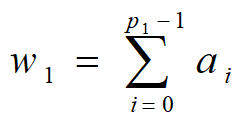#### 2.4.5 Trivium算法

Trivium算法是一种对称密钥同步序列密码算法。它的设计目的是在计算能力有限的硬件上高效实现安全加密，同时兼顾软件实现效率。 Trivium的标准输入为一个80位的密码和一个80位的起始向量（IV）。和大部分同步序列密码算法一样，Trivium的核心组件是一个密码学安全的伪随机数生成器（CSPRNG）。通过将密码和起始向量加载到该伪随机数生成器中，Trivium算法将计算出所需的密钥流。然后，通过将明文位依次与密文位进行异或操作，计算并输出密文。 Trivium伪随机数生成器可以看作由三个线性反馈移位寄存器组成。它们的长度分别是93、84和111位。寄存器和寄存器之间通过非线性逻辑连接。

• 将80位的密钥加载到寄存器A的左边，并将寄存器A的剩余位以0填充。
• 将80位的IV加载到寄存器B的左边，并将寄存器B的剩余位以0填充。
• 将寄存器C的最后三位以1填充，并将寄存器C的剩余位以0填充。
• 重复1152次“下一状态算法”中的操作，同时并不输出密钥。

### 常见的流密码

#### 2.5.1 A5/1

A5 / 1是用于在GSM蜂窝电话标准中提供无线通信隐私的流密码。它是为GSM使用指定的七种算法之一。最初是保密的，但通过泄漏和逆向工程成为公众的知识。 密码中存在一些严重的弱点。 A5/1算法使用3个线性反馈移位寄存器，简称为LFSR。三个寄存器的分别为19位、22位、23位。这里要使用三个共包含64位二进制的线性反馈移位寄存器，这个设计绝非偶然。A5/1算法的密钥K也是64位。该密钥用于三个线性反馈移位寄存器的初始填充，也就是说，该密钥用于充当三个寄存器的初始值。这三个寄存器用密钥填充之后，就可以开始生成密钥流了。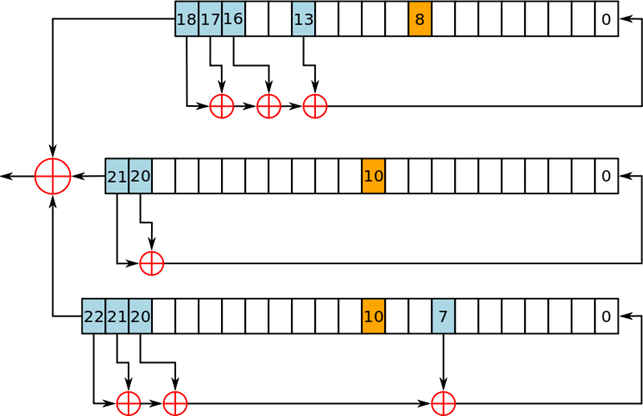A5 / 1流密码使用三个LFSR。如果一个寄存器的时钟位（橙色）与其他两个寄存器中的一个或两个的时钟位一致，则该寄存器会被时钟控制。

A5 / 1的每个回合步骤可以拆分为：取KEY，反馈多项式（Feedback Polynomial），位移

1. 取Key： 取三个寄存器的最后一位，也就是19位、22位、23位，进行XOR操作，得到的结果为这一轮Key的一位。
2. 反馈多项式（Feedback Polynomial）： LFSR进行位移的前置操作，具体可以分成两步：判定是否需要进行位移，判定新的充填的值。 判定是否需要进行位移： 这里使用的是择多原则，三个寄存器中选择三个钟控信号，19位中的第8位，22位，23位中皆为第10位，两种二进制0,1，必定有0或1数量居多，居多的寄存器需要进行位移操作。 判定新的充填的值： 这里需要用到反馈多项式：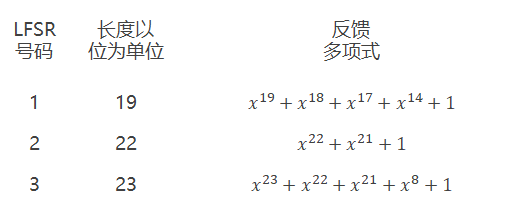XOR后得到的值是充填到第0位的值。
3. 位移： 三个寄存器，由上一步的判定后进行位移，空出第0位用于充填反馈多项式得到的值。

A5/1的安全性 在欧洲和美国使用A5 / 1 。A5 / 2是对某些出口地区的算法的有意削弱。A5 / 1是在1987年开发的，当时GSM尚未考虑在欧洲以外使用，而A5 / 2是在1989年开发的。虽然两者最初都保密，但总体设计在1994年泄露，算法完全在1999年由Marc Briceno从GSM电话机中逆向工程。2000年，大约1.3亿GSM客户依靠A5 / 1来保护其语音通信的机密性; 到2014年为72亿。 爱德华斯诺登在2013年泄露的文件称，NSA“可以处理加密的A5 / 1”。

#### 2.5.2 RC4算法

• 密钥调度算法（KSA） 密钥调度算法被用于初始化“S”盒，“密钥长度”被定义为密钥中的字节数，并且可以在1≤keylength≤256的范围内，通常在5和16比特之间，对应于密钥长度为40-128 位。

• 初始化长度为256的S盒。第一个for循环将0到255的互不重复的元素装入S盒。第二个for循环根据密钥打乱S盒。

for i from 0 to 255
S[i] := i
end for
j := 0

for( i=0 ; i<256 ; i++)
j := (j + S[i] + key[i mod keylength]) % 256
swap values of S[i] and S[j]
end for


i := 0
j := 0

while GeneratingOutput:
i := (i + 1) mod 256   //a
j := (j + S[i]) mod 256 //b
swap values of S[i] and S[j]  //c
k := inputByte ^ S[(S[i] + S[j]) % 256]
output K
endwhile


#### 2.5.4 破译RC4算法

CR4被广泛用于常用协议中，包括有线等效保密（WEP），用于无线网络的安全算法，以及HTTPS的安全套接字层（SSL）和传输层安全（TLS）协议；事实上，在所有TLS流量中，约有50%的流量目前在使用RC4算法来保护。 一个256位的RC4密码共有256！种可能，即$2^{1600}$ 种可能，这样就使得穷举攻击变得不可能。 但是RC4算法不可能从根本上模拟随机数，因此仍然存在一定的弱点。例如在第一阶段KSA中，KSA永远不会生成某类密钥，例如：$j=j+1$与$S(j)=1$。事实证明这类密钥的数量是所有可能密钥数的2^(−2n)。在256位中（28），就是256/2^16 。 40比特的RC4密码曾被广泛使用在WEP中，然而攻击者通过已知明文攻击，借助字典在几分钟之内进行快速破解。

### 小结

• 针对软件设计：CR4、SEAL等
• 针对硬件设计：基于LFSR算法的A5/1、A5/2、Trivium等 在过去的许多年里，许多流密码已经被认为安全性未知，在一些安全性要求比较高的地方不适合使用。 但流密码仍然具有很多天然优势，尤其是一些存储空间有限、功耗要求苛刻、性能羸弱的硬件应用程序中，流密码仍然被广泛使用。 即使一个伪随机数生成器生成的随机数具有良好的统计学属性，但不一定适用于加密。